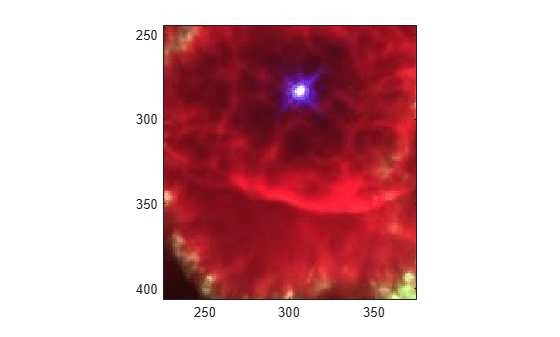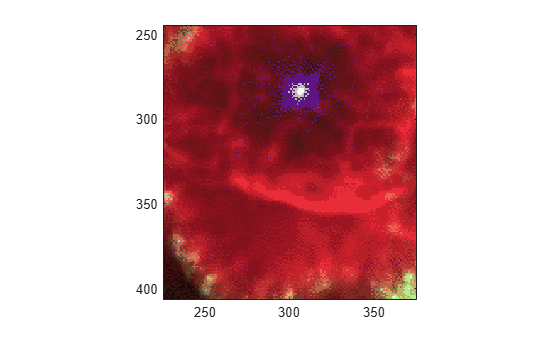# rgb2ind

Convert RGB image to indexed image

## Syntax

``[X,cmap] = rgb2ind(RGB,Q)``
``[X,cmap] = rgb2ind(RGB,tol)``
``X = rgb2ind(RGB,inmap)``
``___ = rgb2ind(___,dithering)``

## Description

example

````[X,cmap] = rgb2ind(RGB,Q)` converts the RGB image to an indexed image `X` with associated colormap `cmap` using minimum variance quantization with `Q` quantized colors and dithering.```
````[X,cmap] = rgb2ind(RGB,tol)` converts the RGB image to an indexed image using uniform quantization with tolerance `tol` and dithering.```
````X = rgb2ind(RGB,inmap)` converts the RGB image to an indexed image using the inverse colormap algorithm with specified colormap `inmap` and dithering.```
````___ = rgb2ind(___,dithering)` enables or disables dithering.```

## Examples

collapse all

Read and display a truecolor `uint8` JPEG image of a nebula.

```RGB = imread('ngc6543a.jpg'); figure imagesc(RGB) axis image zoom(4)```Convert RGB to an indexed image with 32 colors.

```[IND,map] = rgb2ind(RGB,32); figure imagesc(IND) colormap(map) axis image zoom(4)```## Input Arguments

collapse all

RGB image, specified as an m-by-n-by-3 array.

Data Types: `single` | `double` | `uint8` | `uint16`

Number of quantized colors used for minimum variance quantization, specified as a positive integer that is less than or equal to 65,536. The returned colormap `cmap` has `Q` or fewer colors.

Tolerance used for uniform quantization, specified as a number in the range [0, 1]. The returned colormap `cmap` has `(floor(1/tol)+1)^3` or fewer colors.

Input colormap, specified as a c-by-3 matrix with values in the range [0, 1]. Each row of `inmap` is a three-element RGB triplet that specifies the red, green, and blue components of a single color of the colormap. The colormap has a maximum of 65,536 colors.

Data Types: `double`

Perform dithering, specified as `'dither'` or `'nodither'`. Dithering increases the color resolution at the expense of spatial resolution. For more information, see `dither`.

If you select `'nodither'`, then `rgb2ind` does not perform dithering. Instead, the function maps each color in the original image to the closest color in the new colormap.

## Output Arguments

collapse all

Indexed image, returned as an m-by-n matrix of nonnegative integers. If the length of `map` is less than or equal to 256, then the output image is of class `uint8`. Otherwise, the output image is of class `uint16`. The value `0` in the output array `X` corresponds to the first color in the colormap.

### Note

The values in image `X` are indexes into the colormap `map` and should not be used in mathematical processing, such as filtering operations.

Data Types: `uint8` | `uint16`

Colormap, returned as a c-by-3 matrix with values in the range [0, 1]. Each row of `cmap` is a three-element RGB triplet that specifies the red, green, and blue components of a single color of the colormap. The colormap has a maximum of 65,536 colors.

Data Types: `double`

## Algorithms

• Uniform Quantization — If you specify `tol`, then `rgb2ind` uses uniform quantization to convert the image. Uniform quantization cuts the RGB color cube into smaller cubes of length `tol`. For example, if you specify a `tol` of 0.1, then the edges of the cubes are one-tenth the length of the RGB cube. The total number of small cubes is:

```t = (floor(1/tol)+1)^3 ```

Each cube represents a single color in the output image. Therefore, `t` is the maximum length of the colormap . `rgb2ind` removes any colors that don’t appear in the input image, so the actual colormap can be smaller than `t`.

• Minimum Variance Quantization — If you specify `Q`, then `rgb2ind` uses minimum variance quantization. Minimum variance quantization cuts the RGB color cube into smaller boxes (not necessarily cubes) of different sizes, depending on how the colors are distributed in the image. If the input image actually uses fewer colors than the number specified, then the output colormap is also smaller.

• Inverse Colormap — If you specify an input colormap `inmap`, then `rgb2ind` uses colormap mapping. The inverse colormap algorithm quantizes the specified colormap into 32 distinct levels per color component. Then, for each pixel in the input image, the closest color in the quantized colormap is found.

 Spencer W. Thomas, "Efficient Inverse Color Map Computation", Graphics Gems II, (ed. James Arvo), Academic Press: Boston. 1991. (includes source code)